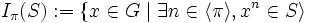# Isolator

## Definition

Suppose$G$ is a group (not necessarily finite),$S$ is a subset of$G$, and$\pi$ is a set of primes. Then, the isolator of$S$ at the set$\pi$, denoted$I_\pi(S)$, is defined as:$I_\pi(S) := \{ x \in G \mid \exists n \in \langle \pi \rangle, x^n \in S \rangle$

where$n \in \langle \pi \rangle$ means that$n$ is a$\pi$-number, i.e. all prime divisors of$n$ are in$\pi$.

## Facts

In a nilpotent group, the isolator of any subgroup, with respect to any set of primes, is nilpotent. For full proof, refer: Isolator of subgroup is subgroup in nilpotent group